Courses
Courses for Kids
Free study material
Free LIVE classes
More

# Convex and Concave Lenses - JEELIVE
Join Vedantu’s FREE Mastercalss

## How Images are Formed by Lenses?

As we all know, a ray of light from an object gets refracted by a lens based on the principle of refraction of light. The first law of refraction is that the incident ray, refracted ray, and the normal to the interface of two mediums all lie in the same plane. The second law of refraction is that the ratio of sine of angle of incidence to the sine of angle of refraction is constant. These two laws are followed by all the rays which get refracted through any surface. Refraction mainly happens when a ray of light moves from one medium to another medium.Refraction of ray of Light

We are also aware that there are two types of spherical surfaces or lenses – convex lens and concave lens. A convex lens is also called a converging lens, whereas a concave lens is also called a diverging lens. In this article, we are going to learn about the image formed by a convex and concave lens for an object placed at different positions.

## Spherical Lens

The lenses which are curved from both sides and look like two arcs are joined to form one lens are termed as spherical lenses. Spherical lens are broadly classified into two types:

1. Concave or divergent lens

2. Convex or convergent lens

## Lens Diagram

The diagram of spherical lenses - convex lens and a concave lens is given below: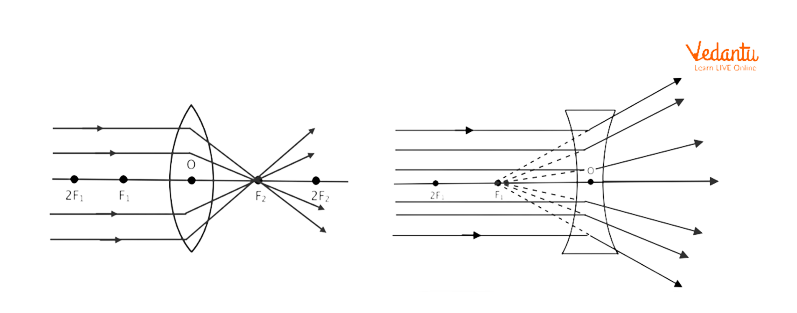Lens Diagram of Concave and Convex Lens

## What is a Convex Lens?

A lens that converges all the rays of light from an object, coming parallel to its principal axis is known as a convex lens. Due to its converging property, convex lenses are also called converging lenses. It is made by joining two spherical surfaces which are thin at the edges and thick at the centre.Convex Lens

## Image Formation by Convex Lens at Different Positions of Object

If an object is placed at a different position in front of a convex lens, different images are formed. Let’s understand the images formed by convex lenses at different positions of objects with the following illustration and examples.

There are 6 cases based on the different positions of objects kept in front of the convex lens.

Case -1: When an object is placed at an infinite distance or infinity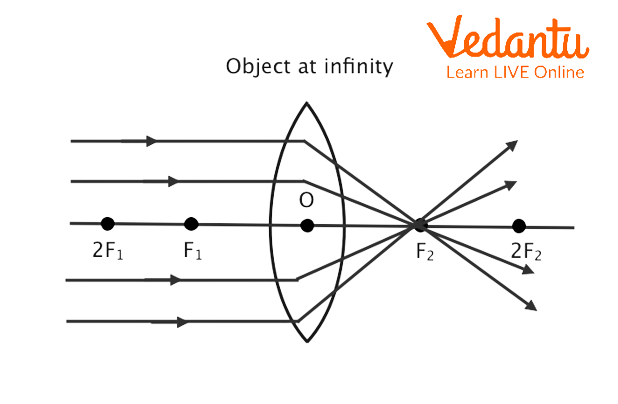When an Object is Placed at Infinite Distance or Infinity From a Convex Lens

When an object is placed at an infinite distance from the convex lens, its rays are parallel to the principal axis and after refraction meets at the focus. Thus, the image of an object placed at an infinite distance from the convex lens is produced at focus as depicted above. The image is real, inverted, and smaller than the size of the object.

Case -2: When an object is placed beyond 2F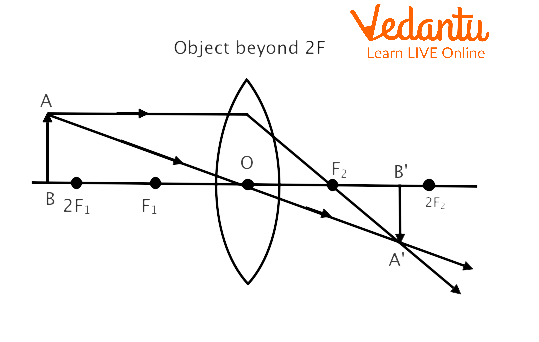When an Object is Placed beyond 2F from a Convex Lens

When an object is placed beyond 2F, some rays are parallel to the principal axis and some pass through the optical centre. After refraction, the rays parallel to the principal axis pass through focus. The rays that pass from the optical centre and the rays passing from the focus meet at the point A. Thus, the image of an object placed beyond 2F of the convex lens is produced at point A. The image is real, inverted, and smaller than the size of the object.

Case -3: When an object is placed at 2F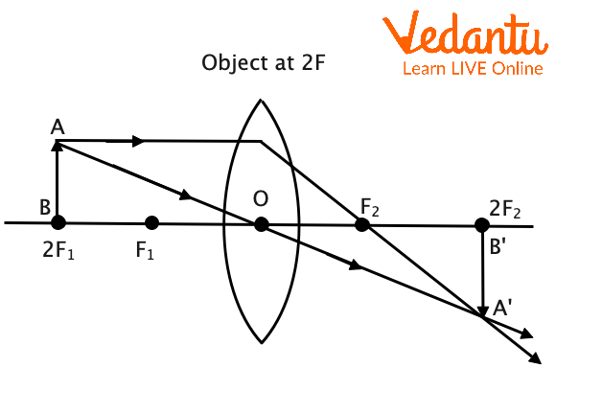When an Object is Placed at 2F

When an object is placed beyond 2F, some rays are parallel to the principal axis and some pass through the optical centre. After refraction, the rays parallel to the principal axis pass through focus. The rays that pass from the optical centre and the rays passing from the focus meet at the point A which is 2F2. Thus, the image of an object placed at 2F of the convex lens is produced at 2F2. The image is real, inverted, and the same as the size of the object.

Case -4: When an object is placed between F and 2F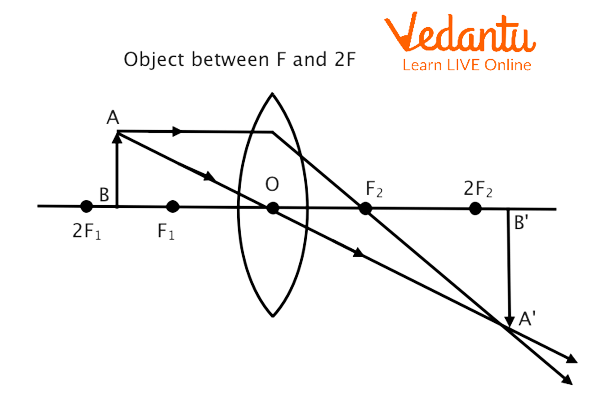When an Object is Placed Between F and 2F

When an object is placed between F and 2F, some rays are parallel to the principal axis and some pass through the optical centre. After refraction, the rays parallel to the principal axis pass through focus. The rays that pass from the optical centre and the rays passing from the focus meet at the point A which is beyond 2F2. Thus, the image of an object placed between F and 2F of the convex lens is produced beyond 2F2. The image is real, inverted, and larger than the size of the object.

Case -5: When an object is placed at F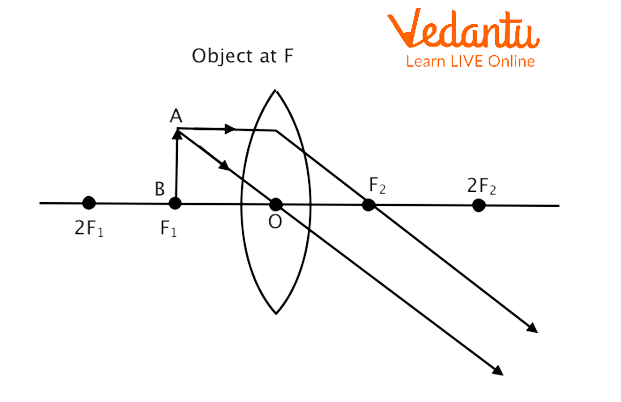When an object is placed at F

When an object is placed at F, some rays are parallel to the principal axis and some pass through the optical centre. After refraction, the rays parallel to the principal axis pass through focus. The rays that pass from the optical centre and the rays passing from the focus do not meet as they become parallel to each other. Thus, the image of an object placed at F of the convex lens is produced at infinity. The image is real, inverted, and larger than the size of the object.

Case -6: When an object is placed between O and F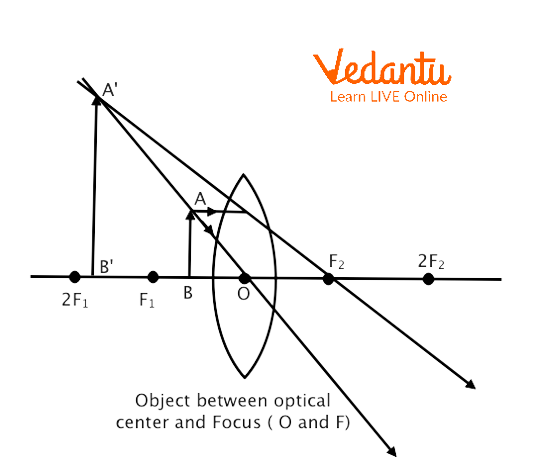When an object is placed between O and F

When an object is placed between O and F, some rays are parallel to the principal axis and some pass through the optical centre. After refraction, the rays parallel to the principal axis pass through focus. The rays that pass from the optical centre and the rays passing from the focus do not meet as they are diverging to each other. Thus, on extending both the rays behind the lens, the image of an object placed at F of the convex lens is produced at A which is beyond F. The image is virtual, erect, and larger than the size of the object.

## Convex Lens Image Formation Table

The image formation table of the convex lens is given below:

 Object Image Size of Image Nature of Image At infinity At focus F2 Highly diminished and point size Real and inverted At 2F1 At 2F2 Same size Real and inverted At focus F1 At infinity Infinitely large Real and inverted Between focus F1 and optical centre Same size Enlarge Virtual and erect Between F1 and 2F1 Beyond 2F2 Enlarge Real and inverted Beyond 2F1 Between F2 and 2F2 Diminished Real and inverted

## What is a Concave Lens?

A lens which diverges all the rays of light from an object, coming parallel to its principle axis, is known as a concave lens. Due to its diverging property, concave lenses are also called diverging lenses. It is made by joining two spherical surfaces which are thin at the centre and thick at the edges.Concave Lens

## Image Formed By Concave Lens

As we know that the concave lens is thicker at the edges and thinner at the centre, it diverges all the rays which fall on it. Thus, none of the rays intersect after the refraction and hence the image formed by concave lens is virtual in nature.

## Image Formation by Concave Lens at Different Positions of Object

If an object is placed at a different position in front of a concave lens, different images are formed. Let’s understand the images formed by concave lenses at different positions of objects with the following ray diagrams of concave lenses.

There are 2 cases based on the different positions of objects kept in front of the concave lens.

Case -1: When an object is placed at infinite distance or infinity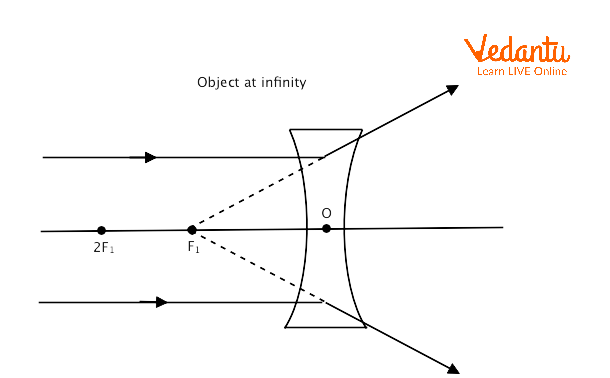When an Object is placed at Infinite Distance or Infinity

When an object is placed at an infinite distance from the concave lens, its rays are parallel to the principal axis and after refraction, they appear to meet at the focus as they are diverging. Thus, the image of an object placed at an infinite distance from the concave lens is produced at focus as depicted above. The image is virtual, erect, and smaller than the size of the object.

Case-2: When an object is placed between infinity and the optical centre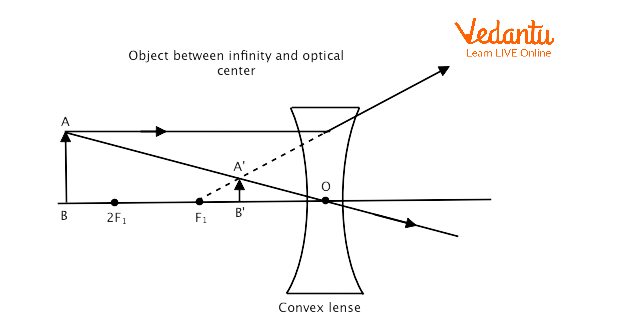When an Object is placed Between Infinity and the Optical Centre

When an object is placed between infinity and the optical centre, some rays are parallel to the principal axis and some pass through the optical centre. After refraction, they diverge. Thus, on extending the ray parallel to the principal axis, the image of an object placed between infinity and the optical centre of the concave lens is produced at point A as depicted above. The image is formed behind the mirror, erect, and smaller than the size of the object.

## Summary

A lens which converges all the rays of light from an object, coming parallel to its principle axis, is known as a convex lens. Due to their converging property, convex lenses are also called converging lenses. It is made by joining two spherical surfaces which are thin at the edges and thick at the centre.

A lens which diverges all the rays of light from an object, coming parallel to its principal axis, is known as a concave lens. Due to their diverging property, concave lenses are also called diverging lenses. It is made by joining two spherical surfaces which are thin at the centre and thick at the edges. There are 6 cases based on the different positions of objects kept in front of the convex lens and 2 cases based on the different positions of objects kept in front of the concave lens, which are explained in the article.

Last updated date: 20th Sep 2023
Total views: 120k
Views today: 4.20k

## FAQs on Convex and Concave Lenses - JEE

1. What is a convex and concave lens?

A lens which converges all the rays of light from an object, coming parallel to its principle axis, is known as a convex lens. Due to their converging property, convex lenses are also called converging lenses. It is made by joining two spherical surfaces which are thin at the edges and thick at the centre. A lens which diverges all the rays of light from an object, coming parallel to its principal axis, is known as a concave lens. Due to their diverging property, concave lenses are also called diverging lenses.

2. Which type of image is formed by a concave lens on the screen?

A concave lens always produces a virtual, erect, and reduced picture. Depending on how close an object is to a concave mirror, the picture it creates can be physical or virtual, magnified or shrunk, and upright or inverted. A true image is one that can be obtained on a screen.